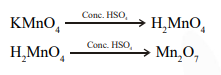# When a small amount of

Question:

When a small amount of $\mathrm{KMnO}_{4}$ is added to concentrated $\mathrm{H}_{2} \mathrm{SO}_{4}$, a green oily compound is obtained which is highly explosive in nature. Compound may be:

1. $\mathrm{Mn}_{2} \mathrm{O}_{3}$

2. $\mathrm{MnSO}_{4}$

3. $\mathrm{Mn}_{2} \mathrm{O}_{7}$

4. $\mathrm{MnO}_{2}$

Correct Option:

Solution: